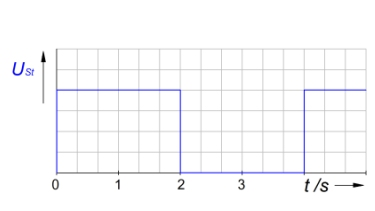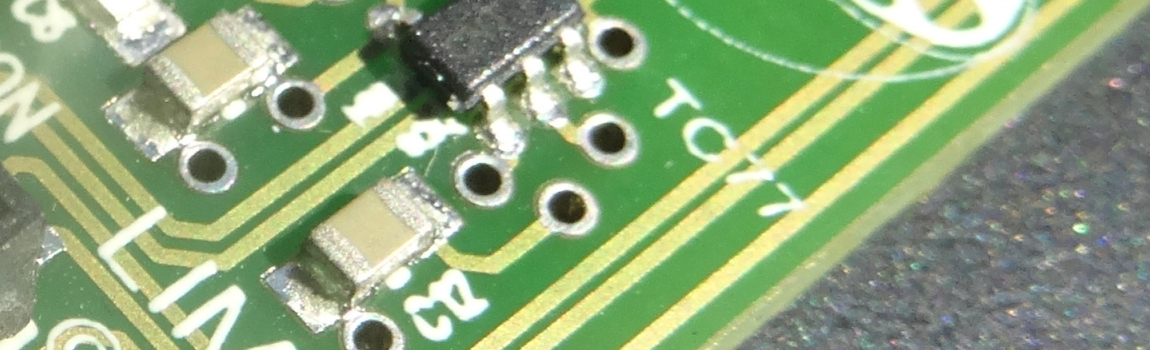In depandance of their capacity, capacitors can store electric energy. They can be charged and discharged, so the charge varies with time.

1 Charging and discharging a capacitorCapacitors are one of most components of electric circuits. Every IC stabilizes the voltage with it. You will learn how capacitors work and why they are capable to stabilize voltage. Further you will learn about parallel and series combinations and how the charge varies with time.

Capacitors have the ability to store energy. Two leads connect two plates which are se­parated by a dielectric. Even the two plates are isolated, there will flow a current if you apply a p.d. across the two leads. But only for a very short moment.

Electrons will move from the negative terminal toward one plate and from the other plate toward the positive terminal. So one plate gets charged negative and the other positive. The reason? Different charges attract, identical repel.

2 Capacitance and construction of a capacitorThe larger the capacitance, the more charge the capacitor stores for each volt applied:

$C=\frac{Q}{V}$

Every capacitor has a fixed capacity. The capacity C depends on design. This means, the larger the area A, the smaller the distance of separation of the two plates d and the better the dielectric, the higher the capacity.

$C=\epsilon _0 \cdot \epsilon_r \cdot \frac{A}{d}$ $~~~~~~~~$ $\epsilon_0=8,85\cdot 10^{-12}\frac{As}{Vm}$ $~~~~~$ $[C]=\frac{As}{Vm}\cdot \frac{m^2}{m}=F$

$\epsilon_0$ is the permittivity of free space. Any other insulating material beside air and vacuum has a permittivity $\epsilon_r$ greater than 1.

3 Parallel combinations of capacitors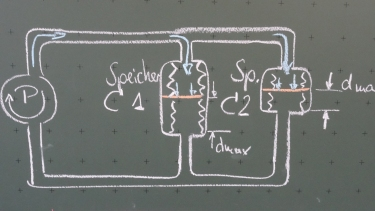A parallel combination of capacitors means enlarging the capacity.

\begin{align*} I &= I_1 + I_2 ~~~|I=Qt\\ Q &= Q_1 + Q_2 ~~~|Q=C\cdot V\\ CV&= C_1V_1 + C_2V_2 ~~~|V=V_1=V_2\\ C &= C_1+C_2\\ \end{align*}

Note: Every capacitor has a maximum voltage. In parallel the maximum voltage will be determined by the lowest.

4 Capacitors in series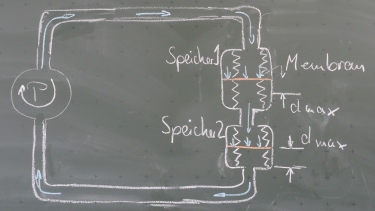Capacitors in series means that the over all capacity is smaller then the smallest single capacitor.

\begin{align*} V &= V_1 + V_2 ~~~|V=Q/C\\ \frac{Q}{C} &= \frac{Q_1}{C_1} + \frac{Q_2}{C_2} ~~~|Q=Q_1=Q_2\\ \frac{1}{C} &= \frac{1}{C_1} + \frac{1}{C_2}\\ \end{align*}

Note: The maximum voltage increases with every additional capacitor.

5 Charging a capacitor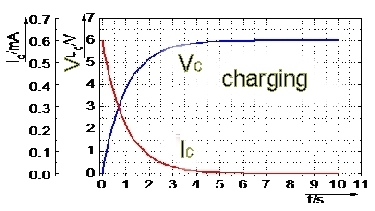Charging a capacitor will result in an exponential function for voltage and current.

$$V_c=V_0(1-e^{-\frac{t}{\tau}})$$ $$I_c=\frac{V_0}{R}e^{-\frac{t}{\tau}}$$

The time constant $\tau=RC$ is the remaining time until the capacitor is charged 63 % of input voltage.

6 Discharging a capacitor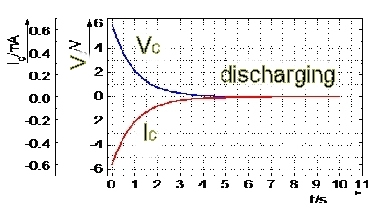Discharging a capacitor will result in an exponential function as well. The current must be negative, since charges have to flow back the same way they came.

$$V_c=V_0e^{-\frac{t}{\tau}}$$ $$i_c=-\frac{V_0}{R}e^{-\frac{t}{\tau}}$$

#### Question 1 Construction of a capacitor and Capacity

by J. Blum, CDSC, Thailand

1. Explain how a capacitor is built and what it can be used for.
2. Explain why an initial current flows while charging. When does it stop?

Two circular parallel metal plates with the radius r = 24 cm, are arranged in a distance d = 1.2 mm as a plate capacitor. The applied voltage is 240 V.

1. Calculate the capacitance of this arrangement and the stored charge QC.
2. Calculate the electric field strength E between the plates and the stored energy EC in the electric field.
3. The force of attraction between oppositely charged capacitor plates equals $F = 0,5 \cdot E \cdot Q$. Determine the force F, which one plate exerts to the other.
4. Compare the result with the force of the two point charges $Q_C = 316.8~nC$ and $-Q_C$ in the same distance.1. A capacitor is built out of two metal plates (area A) and a certain distance d in between. The material in between is called dielectric.
2. If a voltage is applied electrons repell each other and move to the one side of the capacitor ...

3. capacitance: $C=\epsilon \frac{A}{d}=1.32~nF$

charge: $Q_C=VC=316.8~nC$

4. Electric field strength: $E=\frac{E}{d}=200~000~V/m$

Energy: $E_{el}=\frac{1}{2}CV^2=38.02\cdot 10^{-6}J$

5. Force of attraction between plates: $F= 0,5 \cdot E \cdot Q=31.68~mN$
6. Force of two point charges: $F=k\cdot \frac{Q_1 \cdot Q_2}{d^2}=626,69~N$

#### Question 2 Capacitors in series and parallel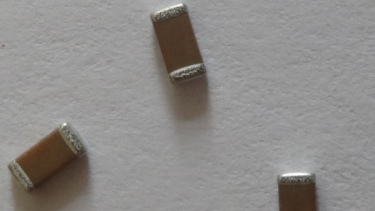Knut has bought capacitors by Conrad electronics with value 10 µF/25 V.

1. How does he has to connect six capacitors in order to get the maximum capacity? Calculate the capacitance and the maximum voltage.
2. How does he has to connect six capacitors in order to get the maximum voltage? Calculate the the maximum voltage and capacitance.
3. Design a circuit for 20 µF and 50 V
4. Which advantage have electrolytic capacitors in comparision with ceramic capacitors?
1. In parallel: $C=6 \cdot 10~\mu F= 60~\mu F$, $V=25~V$

2. In series: $V=6 \cdot 25~V=150~V$, $C=1/(1/C_1+~...~+1/C_6)$$=1,67~\mu F 3. Respectively 2 in series (50~V and 5~\mu F)and then 4 times parallel. 4. Beside a high capacity, electrolytic capacitors have a high maximum voltage. #### Question 3 charging a capacitor A capacitor with 47 µF is connected to 24 V with a series resistance of 2,1 kΩ. 1. How high is the time constant and how long does charging last? 2. Calculate the capacitor's voltage after 1.5 τ. 3. After which time the voltage reaches 90 % of its maximum? 4. How high is the maximum inrush current? 5. After which time the current is down to 30 % of its maximum value? 6. Draw the t-VC- und t-IC-diagram. Plot all calculated values.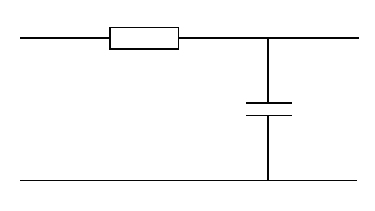1. time conatant: \tau =R\cdot C = 98,7~ms charging time: 5\tau =493,5~ms 2. time: 2\tau=197,4~ms capacitors voltage: V_C(1,5\tau)=V_0(1-e^{-t/\tau})=$$24~V(1-e^{-1,5\tau /\tau})=18,64~V$

3. questioned voltage: $V_C=90\% \cdot V_0=21,6~V$

$V_0(1-e^{-t/\tau})=V_C~~|:V_0$

$1-e^{-t/\tau}=V_C/V_0~~|-1 ~|\cdot(-1)$

$e^{-t/\tau}=1-V_C/V_0~~|ln(...)$

$-t/\tau=ln(1-V_C/V_0)~~|\cdot (-\tau)$

$t=-\tau\cdot ln(1-V_C/V_0)~~|\text{insert}$

$t=227~ms$

4. inrush current: $I_0=V_0/R=24~V/2,1~k\Omega=$$11,42~mA$

5. questioned current: $I_C=30\% \cdot I_0=3,426~mA$

$V_0/R\cdot e^{-t/\tau})=I_C~~|\cdot (-R/V_0)$

$e^{-t/\tau})=I_C\cdot R/V_0~~||ln(...)$

$-t/\tau=ln(I_C\cdot R/V_0)~~|\cdot (-\tau)$

$t=-\tau\cdot ln(I_C\cdot R/V_0)~~|\text{insert}$

$t=118,91~ms$

6. diagram:#### Quiz Energy storage

idea from studentsA 1 kΩ load is supplied with 325 V. After power failure a capacitor shall supply the circuit for 5 s with at least 63,3 % the input voltage.

Which capacity is necessary?

Calculate the voltage: $\frac{100~\%}{325~V}=\frac{63,3~\%}{V_C}$, $V_C=205,725~V$

Calculate the time constant: $\tau=\frac{t}{-ln(V_C/V_0)}=10,934~s$

Calculate the capacitance: $C=\tau /R=10,934~mF$

Alternatively estimation out of diagram: $0,633 ~\hat{=}~ 0,5~\tau$

#### Question 4 signal change

A RC-circuit is supplied with the following control voltage. Plot the signal for voltage and current,

1. when the charging time of the capacitor is 1 s.
2. when the charging time of the capacitor is 2 s.
3. when the charging time of the capacitor is 4 s.